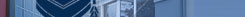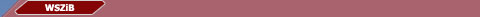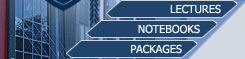1 Introduction to Simulation and Modeling 1.4 Model Execution: Event driven versus Time driven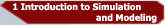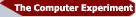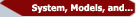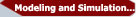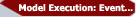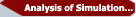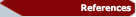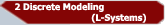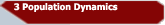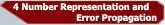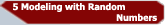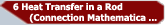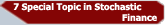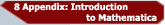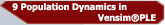We have seen that in continuous systems the state variables change continuously with respect to time, whereas in discrete systems the state variables change instantaneously  at separate points in time. Unfortunately for the computational experimentation there are but a few systems that are either completely discrete or completely continuous state, although often one type dominates the other in such hybrid systems. The challenge here is to find a computational model that mimics closely the behaviour of the system, specifically the simulation time-advance approach is critical.
If we take a closer look into the dynamic nature of simulation models, keeping track of the simulation time as the simulation proceeds, we can distinguish between two time-advance approaches: time-driven and event-driven.

Time-driven   Simulation
For continuous systems, time-driven  simulations  advance time with a fixed increment. With this approach the simulation  clock is advanced in increments of exactly Δt time units. Then after each update of the clock, the state variables are updated for the time interval [t, t+Δt]. This is the most widely known approach in simulation of natural systems.
Less widely used is the time-driven  paradigm applied to discrete systems. In this case we have specifically to consider whether:
• The time step Δt is small enough to capture every event in the discrete system. This might imply that we need to make Δt arbitrarily small, which is certainly not acceptable with respect to the computational times involved.
• The precision required can be obtained more efficiently through the event-driven execution mechanism. This primarily means that we have to trade efficiency for precision.

Event-driven  Simulation
In event-driven  simulation the next-event time advance approach is used. For  the case of discrete systems this  method consists of the following  phases:

Step 1: The simulation clock is initialised to zero and the times of occurrence of future events are determined.
Step 2: The simulation  clock is advanced to the time of the occurrence of the most imminent (i.e. first) of the future events.
Step 3: The state of the system is updated to account for the fact that  an event has occurred.
Step 4: Knowledge of the times of occurrence of future  events is updated and the first step is repeated.

The advantage of this approach is that periods of inactivity  can be skipped over by jumping the clock from event time to the next event time. This is perfectly safe since by definition all state changes only occur at event times. Therefore causality is guaranteed. The event-driven approach to discrete systems is usually exploited in queuing and optimisation problems. However, as we will  see next, it is often also a very interesting paradigm for the simulation of continuous systems.
Consider a continuous system where every now and then (possibly at irregular or probabilistic time steps) discontinuities  occur, for instance in the temperature of a room where the heating is regulated  in some feed-back loop: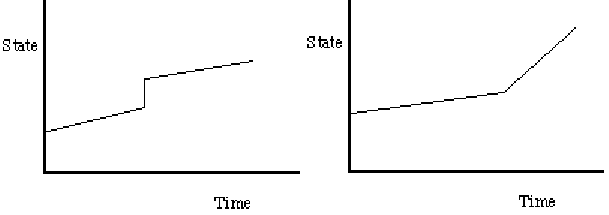Figure 1.8: Typical discontinuities  in Time versus State trajectories of continuous systems or its (higher order) derivative with respect to time.

The difficulty of an efficient time-driven simulation of such a system is in the  integration method applied. Specifically, multi-step  integration  methods to solve the underlying differential equations might prove not to work in this case. The reason is that these methods use extrapolation to estimate the next time step, this would mean that they will try to adapt to the sudden change in state of the system thus reducing the time step to infinite small sizes.
Other examples are:
Continuous Ising spin systems: A configuration is defined by the spin variables s(ν) = ± 1, specified at the vertices ν of a two or three dimensional lattice and an independent Poisson process of attempted spin change arrivals are associated with the vertices of the lattice. If in such a system an attempted  change arrives at vertex ν , the spin s(ν) is changed to -s(ν) with probability P, and with probability 1-P the spins remain unchanged.
Billiard ball models: Used in gas dynamics, where N equal balls  move without  friction in 2D and where each ball moves along a straight line with constant velocity, until  it comes into contact with  another ball. Upon such a contact a collision  occurs where upon the two participating balls instantly  change their velocities and directions.
It is clear that time-driven  simulation might not prove to be acceptable since this mechanism is slow and/or not sufficiently precise if Δt is not chosen to be very small. An event-driven  simulation where the state of the balls is updated from collision to collision of any ball, might prove much more accurate and efficient.
Let's explore this example in a little more detail.

#### I.4.1 Billiard Balls - Example

Consider a Billiard Ball model to simulate the gas dynamics of 4 gas molecules (as long as we do not try to do any physics with this simple system it can perfectly well explain the concepts we are discussing). Assume that the molecules move along the straight line with constant velocity until they hit the wall of a 2D system (kinetic energy is conserved in this model). We can now investigate the consequences of the two paradigms time-driven versus event-driven simulation:

input stop simulation time, Δt;
initialise balls with x,y position and velocity vx,vy in x,y direction;
time = 0.0;
while (time < stop simulation)
{
for each ball do {
x += Δt * vx;
y += Δt * vy;
if ((x == 0)||(x == 1)) vx = -vx;
if ((y == 0)||(y == 1)) vy = -vy;
}
time += Δt;
}

Code 1.2: Pseudo code for time-driven gas simulation.

For  a simulation  time of 1.0 (arbitrary  units) with  a Δt  of 0.001, implying 1000 state evaluations, this time-driven approach (see Table 1.2) results in the following simulated  behaviour of the 4 balls  over time: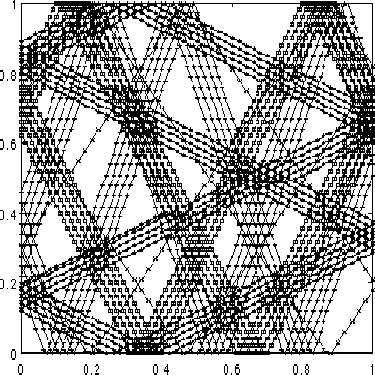Figure 1.9: Time-driven state evaluation of gas model, 1000 state evaluations, 4 molecules.

Next we consider the case of an event-driven simulation of this 4-molecule  gas. The pseudo code shown in Table 1.3 takes into account all the typical aspects of detecting, handling and processing events within the correct time frame.

input stop_simulation_time;
initialise balls with x,y position and velocity vx,vy in x,y direction;
for each ball do {
impact_time = collision_time(ball);
schedule(MOVE, impact_time, ball);
}
prev_time = 0.0;
while (time() < stop_simulation_time) {
next_event(&event, &ball);
switch(event) {
case MOVE:
update_positions(time() - prev_time);
impact_time = collision_time(ball);
schedule(MOVE, impact_time, ball);
prev_time = time();
break;
}
}
collision_time(ball)
{
if (vx > 0) {
t0 = (1 - x) / vx;
}
else {
t0 = -x / xv;
}
if (vy > 0) {
t1 = (1 - y) / vy;
}
else {
t1 = -y / vy;
}
return min(t0, t1);
}
update_positions(Δt)
{
for each ball do {
x += Δt * vx;
y += Δt * vy;
if (x == 0 || x == 1)
vx = -vx;
if (y == 0 || x == 1)
vy = -vy;
}
}

Code 1.3: Pseudo code for an event-driven gas simulation.

For a simulation time of 1.0 (arbitrarily units) with 60 state evaluations, this event-driven approach results in the following simulated behaviour of the 4 balls over time: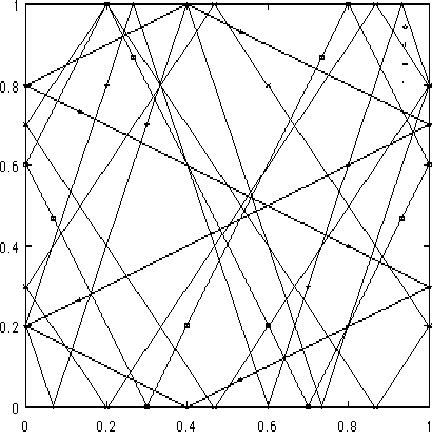Figure 1.10: The trajectory of an event driven billiard ball simulation
From these examples we see that the cumulated error over time in the time-driven simulation, even for a Δt as small as 0.001, results in a violation of the conservation of momentum. The gain we have in event-driven simulations is however partly frustrated by the growth in the (complexity of the) simulation code. If we would like to go to modern (parallel) architecture's, event-driven simulation  becomes increasingly more difficult to code due to the complicated synchronisation protocols. There is always the necessity to find a balance between the pro's and con's of time-driven versus event-driven simulation.
In general we observe that:

•    If the time between the succeeding events becomes small, time driven is preferred (in the example this situation would occur for a system of billions of molecules to be simulated). This is the frequency parameter.
•    The overhead per event (i.e., the state update) is larger with event-driven simulation. This is referred to as the event overhead.
•    The amount of work between any two events plays an important role. This we call the granularity of the system.

The factors: frequency, overhead and granularity, together with the consequences for implementation on modern architecture's and the required accuracy, must be studied carefully before making a decision on what simulation mechanism should be used.

#### I.5 Analysis of Simulation Results

During the development of the simulation model, we must ensure that the model is correctly implemented and that it is representative of the real system. These two steps are called model verification and model validation, respectively. After the model development is complete, the next two issues we will face are those of deciding how many of the initial observations should be discarded to ensure that the model has reached a steady state and how long to run the simulation. These issues are called transient removal and stopping criterion, respectively. These four issues are the main topics of this section.

Lectures | Notebooks | Packages